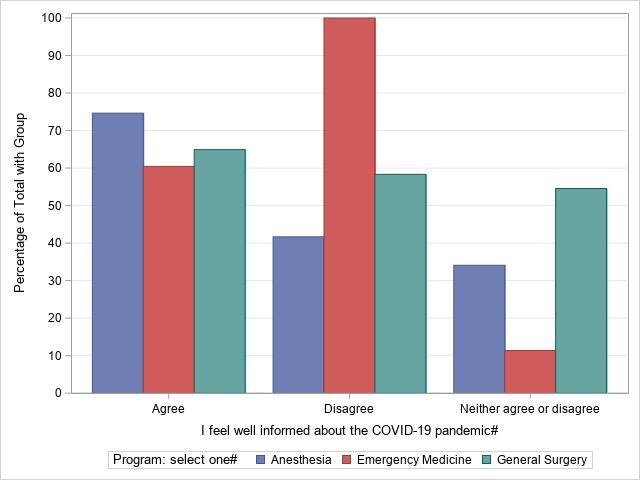## Creating percentage by group in bar chart

Hi,

I am creating a bar chart but would like to get percentage rather than frequency.

And percentage i am interested in is not percentage of total frequency but percentage of group frequency.

My SAS code is like below:

ods graphics on;
proc sgplot data=red.survey2;
run;

And results are attached. I am interested in percentage rather than frequency in y axis.  I a way for the group agree it sums to 100% , for disagree it sums to 100%.

Thanks,

1 ACCEPTED SOLUTION

Accepted Solutions

## Re: Creating percentage by group in bar chart

I suspect your PROC FREQ call is incorrect. You want to get the row totals. The output should look like the following:

``````data Have;
length Informed Program \$8;
input Informed Program Percent;
datalines;
Agree    Anesth  41
Agree    Emerg   26
Agree    GenSurg 33
Disagree Anesth  39
Disagree Emerg    8
Disagree GenSurg 53
Neutral  Anesth  34
Neutral  Emerg   11
Neutral  GenSurg 55
;

proc sgplot data=Have;
vbar Informed / response=Percent group=Program groupdisplay=cluster;
xaxis discreteorder=data;
yaxis grid values=(0 to 100 by 10) label="Percentage of Total with Group";
run;``````
7 REPLIES 7

## Re: Creating percentage by group in bar chart

``vbar I_feel_well_informed_about_the_C /group=Program__select_one_ groupdisplay=cluster stat=percent;``

For future reference, many people will not download attachments, and so the output you want to show us should be pasted directly into your message.

--
Paige Miller

## Re: Creating percentage by group in bar chart

STAT=percent

to the VBAR statement, the Y axis will show percentages.

## Re: Creating percentage by group in bar chart

Hi,

STAT=percent gives me percentage out of total frequency.

I am interested  in getting percentage out of total who agreed, So the sum of 3 bars in agreed should be 100%,

the sum of total who disagreed should be 100% and the sum of neutral should be 100 too.

Thanks,

## Re: Creating percentage by group in bar chart

See the article, "Construct a stacked bar chart in SAS where each bar equals 100%". The article discusses a stacked bar chart, but you can use the same code and change the GROUPDISPLAY= option to CLUSTER

``````
proc sort data=sashelp.cars(where=(Type^="Hybrid")) out=cars;
by Origin;                     /* sort X categories */
run;

proc freq data=cars noprint;
by Origin;                    /* X categories on BY statement */
tables Type / out=FreqOut;    /* Y (stacked groups) on TABLES statement */
run;

title "100% Stacked Bar Chart";
proc sgplot data=FreqOut;
vbar Origin / response=Percent group=Type groupdisplay=cluster;
xaxis discreteorder=data;
yaxis grid values=(0 to 100 by 10) label="Percentage of Total with Group";
run;``````

## Re: Creating percentage by group in bar chart

Thank you very much for reply! I tried the following code;

proc sort data=red.survey2 out=red.informed;
run;
proc freq data=red.informed noprint;
tables Program__select_one_ / out=red.FreqOutt;
run;

proc sgplot data=red.FreqOutt;
vbar I_feel_well_informed_about_the_C / response=Percent group=Program__select_one_ groupdisplay=cluster;
xaxis discreteorder=data;
yaxis grid values=(0 to 100 by 10) label="Percentage of Total with Group";
run; Following is the output.

However, for the group how is sum more than 100%. I wanted it to be 100% for the 3 bars in agree group. similar for disagree and neutral group.

I got the  output-## Re: Creating percentage by group in bar chart

I suspect your PROC FREQ call is incorrect. You want to get the row totals. The output should look like the following:

``````data Have;
length Informed Program \$8;
input Informed Program Percent;
datalines;
Agree    Anesth  41
Agree    Emerg   26
Agree    GenSurg 33
Disagree Anesth  39
Disagree Emerg    8
Disagree GenSurg 53
Neutral  Anesth  34
Neutral  Emerg   11
Neutral  GenSurg 55
;

proc sgplot data=Have;
vbar Informed / response=Percent group=Program groupdisplay=cluster;
xaxis discreteorder=data;
yaxis grid values=(0 to 100 by 10) label="Percentage of Total with Group";
run;``````

## Re: Creating percentage by group in bar chart

Thank you very much! This community is great!!!

Discussion stats
• 7 replies
• 2862 views
• 1 like
• 3 in conversation